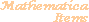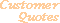Home Older Mathematica Items

Scientific Arts

# Differential Equations for Chemical Kinetics

## Example of Differential Equations for Chemical Kinetics

Chemical reactions of a wide variety can be modeled with coupled (often nonlinear) differential equations. These describe the time evolution of the concentrations of the various chemical species: reactants, intermediaries, catalysts, and products. Such problems are quite simple to set up and solve with Mathematica. The function NDSolve can numerically integrate the differential equations that arise. The resulting concentrations can be plotted as a function of time and also be used to accurately compute the expected concentration of the molecular species.

### Reaction with an intermediate

Here is the reaction,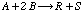where the mechanism of the reaction proceeds through an intermediate species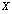.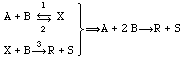The rate equations corresponding to this reaction are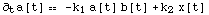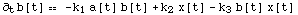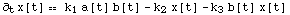where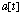,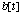, and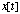represent the concentrations of the species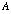,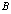, andat the time. The parameters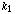,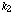, and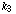(the "rate constants") are considered to be constant here. This might be the case if the reaction proceeds in contact with a heat bath. If the temperature of the mixture of reactants is allowed to be variable, then there needs to be an additional set of equations supplied which model the temperature variation of,, andwith time.

Although these equations do not have an explicit analytic solution, they can be numerically integrated using the built in numerical differential equation solver, NDSolve. Here is the solution for a particular choice of the rate constants,, and. In this example the initial concentrations ofandare equal and that of the intermediaryis zero.

``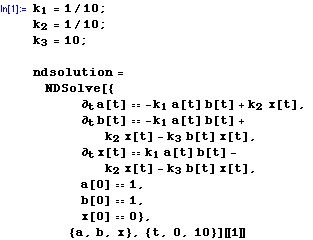``
`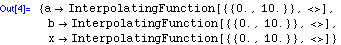`

The result is expressed in terms of a set of replacement rules that give the functions as InterpolatingFunction objects. The notation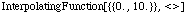, for example, is a shorthand for the numerical information needed by the interpolation algorithm to reproduce the solution.

Here is plot of the result (we multiply the concentration ofby a factor of 50 so that it can be seen in the graph on the same scale asand). The colors of the curves for,, and, are black, red, and green respectively.

``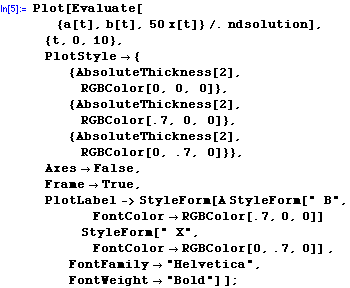``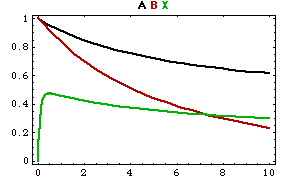For this example, as the reaction proceeds, the intermediary is created and then settles down to an approximately steady state value.

### Reaction with Catalysts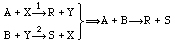The rate equations corresponding to this reaction are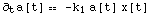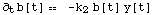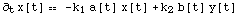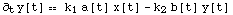Here is a particular case of the integration of these differential equations. The initial concentrations ofandare equal and the rate constantsandchosen as shown.

``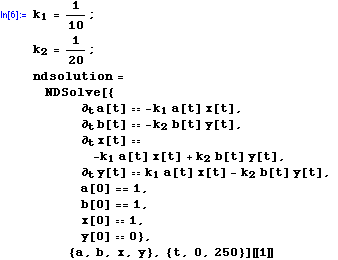``
`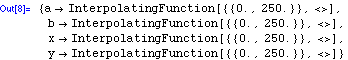`

Here is plot of the result. The colors of the curves for,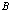,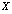, and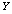are black, red, green, and blue respectively.

``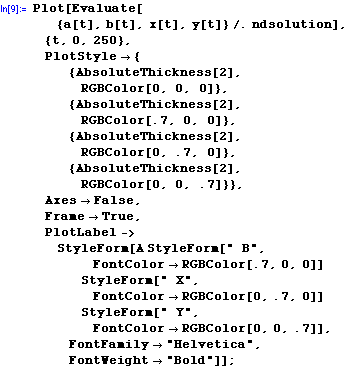``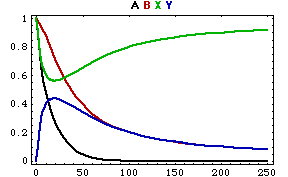It can be seen that, as the reaction proceeds towards completion, the concentrations of the catalysts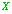and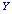eventually return to their initial values.

 For further information on our services send email to info@scientificarts.com . Contents of this web site Copyright © 1999-2011, Scientific Arts, LLC.
 s